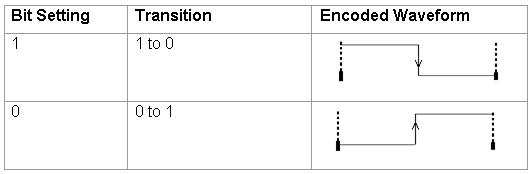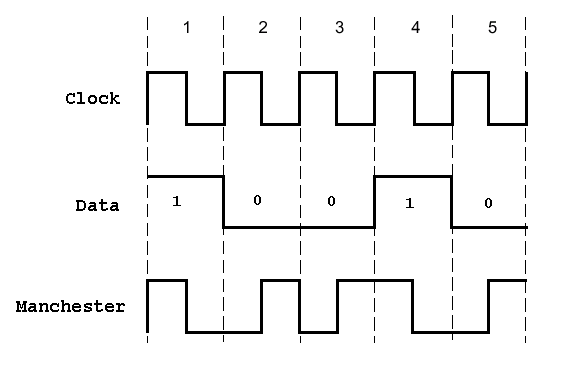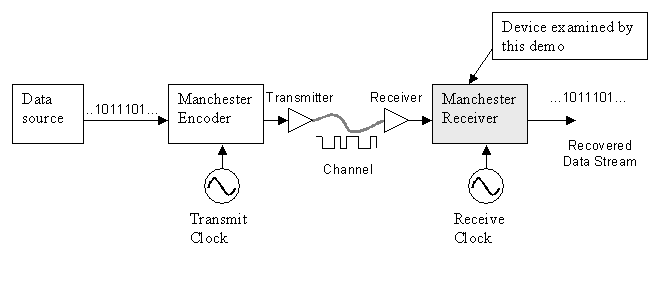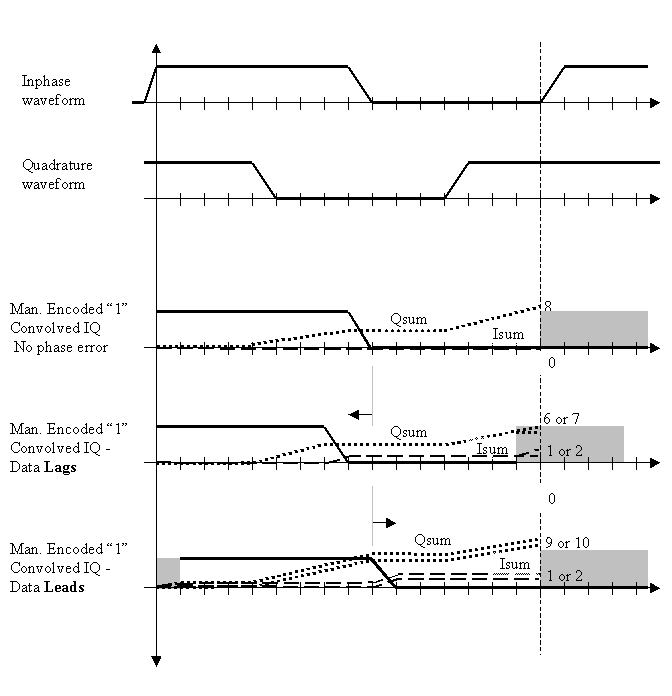The Manchester Receiver example shows how to use the HDL Verifier™ to design, test, and verify a VHDL Manchester Receiver model with clock recovery capabilities.

### Background on Manchester Encoding

Transmission of digital data frequently requires some form of modulation to overcome limits in a physical signal channel. One technique used for modulating digital data is Manchester Encoding. This technique has the following useful characteristics:

• The transmit clock signal can be easily extracted from the received data.

• The encoded signal never produces frequency components near DC, regardless of the data, which is useful for transmission over channels that require AC coupling.

• The encoding circuit is very simple and stateless.

On the negative side, Manchester coding requires substantial bandwidth (above the Shannon limit), which tends to limit its usefulness in wireless applications. However, for connected applications such as short haul Optical fiber and Ethernet, it is frequently a good solution.

The following sections discuss:

### The Encoding

Manchester Encoding involves a transmitter that encodes clock and data signals in a synchronous bit stream, such that each bit represents a signal transition. The following table shows how each bit setting is defined for an encoding:Transitions in the Manchester Encoding occur at the center and beginning of each bit. The transition at the center is defined by the bit value, while the transition at the beginning is dependent on the value of the previous bit. Consider the following diagram:As the preceding Manchester encoded signals shows:

• The value of 1 for the first bit forces a high-to-low transition at the center of that bit.

• The value of 0 for the second bit forces a low-to-high transition at the center of that bit and, because the first bit transitioned from high-to-low, no transition occurs at the start of that bit.

• The value of 0 for the third bit forces a low-to-high transition at the center of that bit and because the second bit transitioned from low-to-high, a high-to-low transition occurs at the start of that bit.

• The value of 1 for the fourth bit forces a high-to-low transition at the center of that bit and, because the third bit transitioned from low-to-high, no transition occurs at the start of that bit.

• The value of 0 for the fifth bit forces a low-to-high transition at the center of that bit and, because the fourth bit transitioned from high-to-low, no transition occurs at the start of that bit.

A device that receives the encoded bit stream is responsible for decoding the bit stream by separating the clock and data information. In most cases, the receiver must retrieve the original data stream by using only the encoded signal. This simplifies the communications channel, but means the receiver must overcome the following:

• Differences between the clock used to encode the signal and the clock in the receiver (see the figure below).

• The two clocks can be close in frequency, but small frequency errors occur.

• The phase between the clocks will be arbitrary.The Manchester Receiver example validates the computations performed by a Manchester Receiver device that is modeled in VHDL and simulated in ModelSim®. Numerous approaches are available for implementing a Manchester receiver. This example uses a Delay Lock Loop (DLL) that requires the receiver to use a clock that is very close in frequency to the transmit clock. This results in a simple clock recovery circuit that has a limited frequency lock range.

The receiver over-samples the received data stream at 16 times the data rate. Thus, the receive clock must have a nominal period of 1/16th the data period. To compensate for minor differences between the transmitting and receiving clocks or drifts in the channel delay, the receiver adjusts its data period by up to one clock cycle (+/-) per data period. Thus, the receiver can use 15, 16 or 17 clock cycles to recover the data encoded from the incoming sampled signal. For example, if the receiver clock is slightly faster then the transmitter clock (frequency error), the receive cycle occasionally needs to add an extra clock cycle to compensate.

Large sudden phase errors, such as those that occur at startup time, require multiple data periods to acquire a good lock on the signal. By limiting the maximum phase correction to 1/16th of the total data period, the receiver can be slow to correct large phase errors.

### Decoding with Inphase and Quadrature Convolution

Decoding a received Manchester signal can occur in several ways, but the approach taken in this example is to consider Manchester Encoding as a digital phase modulation with two symbols: +180 and -180 degrees. By convolving the incoming signal with a reference inphase (I) and quadrature (Q) waveform at the modulation frequency, it is possible to extract the data and retrieve information about any phase errors in the received waveform. After one data cycle, the receiver computes two values (referred to as Isum and Qsum in the VHDL code), which are measurements of the I/Q convolution value. The receiver then decodes the values to predict:

• The original transmitted data value for the cycle

• An estimate of the phase error between the incoming signal and the receiver's data period

A critical aspect of this design is the interpretation of the I/Q convolution values. At the end of a data receive cycle, the receiver translates the I/Q values into an estimate of the transmitted data and phase error. One way to present this information is to show these interpretations as plots versus measured I/Q values.

Data is considered invalid if the measured I and Q are completely ambiguous about the encoded data value.

In a similar way, you can generate an I/Q mapping of the phase correction value in plot format. Such a plot gives a visual representation of the decoding block. In practice, the details of this mapping have strong impact on the stability and performance of the Manchester receiver.

In the ideal case where the receiver is perfectly locked to the incoming waveform, the receive cycle is 16 cycles long and the measured I/Q convolution values are fairly easy to interpret. However, data cycles that are 15 or 17 cycles long create some bias in the measurement of the IQ convolution. It is possible to customize the I/Q measurement during these cycles, but that would increase the size and complexity of the receiver. Instead, the data acquisition cycle is extended or shortened with no change in decoding the resulting values. However, this decoder bias can create problems with dithering or reduced noise immunity.

### VHDL Implementation of a Manchester Receiver

The focus of this example is a VHDL implementation of a Manchester Receiver. Decoding a Manchester encoded signal presents several challenges, the most prominent of which is clock recovery. The clock is embedded in the received signal and must be extracted to reproduce the original data stream. The figure below shows the example design, which is divided into three main sections of VHDL code:

IQ convolver: Samples the received signal and computes the convolution for the inphase (I) and quadrature (Q) waveforms. For each waveform, the computation is implemented as the sum of XOR operations on the sample and decoded waveform received from the state counter.

Decoder: Models a combinatorial circuit that interprets the results of the I/Q convolver.

State counter: Generates the I/Q waveforms that are convolved with received signals, taking into account phase errors (lags and leads), as necessary. The phase of the I/Q generator is adjusted to match the incoming Manchester encoded waveform. To accomplish the necessary adjustment, at the beginning of a new cycle, the state counter checks an adjustment value, adj, and then changes the period of the next I/Q cycle. This adjustment value is limited to adding or removing a single clock period from the 16 periods that are nominally used for an I/Q waveform.The following timing diagram shows an inphase waveform, quadrature waveform, and the convolved results with no phase error, data lags, and data leads.### MATLAB® Models of VHDL Components

The example includes three MATLAB® functions that test the VHDL model. A MATLAB function maps to each of the three VHDL components:

I/Q convolver: Verifies that the VHDL I/Q convolver code computes expected output for a randomly generated stream of samples. The MATLAB function verifies this by computing the convolution for the inphase and quadrature waveforms (I_wf and Q_wf). The computation is implemented as an XOR and accumulation of the binary signals.

Decoder: Displays a plot of the I/Q mapping generated by the decoder for visual verification.

State counter: Generates the inphase and quadrature waveforms. During test benching, this MATLAB function has complete control of signals applied during the simulation, including clock generation, resets, and so on.

### Starting the MATLAB Server

The example starts the MATLAB server, hdldaemon, such that it uses TCP socket communication with a socket port number identified as available by the operating system.

```hdldaemon('socket',0) % Activate MATLAB server to accept foreign VHDL calls ```

The example then calls hdldaemon with the 'status' option to get the assigned port number and store it in portnum for future reference.

```dstat = hdldaemon('status'); portnum = dstat.ipc_id; ```

Both the server and client parts of an application link must use the same port number. Thus, at some point, the example program needs to forward the portnum over to ModelSim.

### Testing the Decoder

The first component of the Manchester Receiver model to be tested is the decoder. The script creates 2 plots of the transfer function of this entity. This test is simply a visualization of the decoder behavior. The example script:

1. Sets a testisdone flag to 0 and displays informational messages.

```global testisdone; testisdone = 0; ```

2. Sets the project directory to a directory that has write access and is suitable for holding a ModelSim project.

```projectdir = pwd; ```

3. Changes the format of the project directory and decoder VHDL file specifications to the UNIX® format, which ModelSim and Tcl use, by replacing backslashes (\) with forward slashes (/).

```unixprojectdir = ['"' strrep(projectdir,'\','/') '"']; unixsrcfile = ['"' ... strrep(fullfile(matlabroot,'examples','hdlverifier','data','decoder.vhd'),'\','/') ... '"']; ```

4. Defines a sequence of Tcl commands to be executed in the context of ModelSim.

```tclcmd = { ['cd ' unixprojectdir ],... 'vlib work',... ['vcom -performdefaultbinding ' unixsrcfile],... 'vsimmatlab work.decoder',... ['matlabtb decoder -mfunc manchester_decoder -socket ',num2str(portnum)],... 'run 3000',... 'quit -f'}; ```

The previous list defines each command:

• Changes to the writable project directory.

• Adjusts the placement of the ModelSim window so it does not obscure the MATLAB window.

• Creates the project library work if it does not already exist.

• Compiles the VHDL file. The example script specifies the 'performdefaultbinding' option to enable default bindings in the event that they have been disabled in the modelsim.ini file.

• Loads an instance of the VHDL entity decoder for MATLAB test benching with the vsimmatlab command. This command is an HDL Verifier extension to the ModelSim command set.

• Initiates a MATLAB test benching session for the loaded instance of entity decoder with the matlabtb command. This command is an extension to the ModelSim command set. The command in the example specifies the entity instance, the MATLAB function that is to test the entity (manchester_decoder.m), and TCP socket communication with socket port portnum. For a link to be established between ModelSim and MATLAB, the value of portnum must match the socket port that was specified when the MATLAB server (hdldaemon) was started.

• Runs the ModelSim simulation for 3000 iterations of the current resolution limit. By default, the simulation runs for 3000 nanoseconds. Quits ModelSim without asking for confirmation.

5. Starts ModelSim for use with the HDL Verifier with a call to vsim.

```vsim('startupfile','decoder.do','tclstart',tclcmd); ```

This command starts ModelSim with a Tcl command script that executes some general-purpose startup commands and then the user-defined commands specified with the 'tclstart' property. The 'startfile' property causes vsim to write the entire startup Tcl command script to decoder.do for future reference or use.

6. Displays informational messages and waits for (manchester_decoder.m) to run to completion.

```disp('Waiting for testing of ''decoder.vhd'' to complete'); disp('(flag from manchester_decoder.m indicates completion)'); while testisdone == 0, pause(0.501); end disp('MATLAB test of decoder.vhd is complete!'); disp('Check the generated plot for results.'); ```

### Testing the I/Q Convolver

Similarly for the I/Q convolver. The example script:

1. Sets a testisdone flag to 0 and displays informational messages.

```testisdone = 0; ```

2. Sets the project directory to a directory that has write access and is suitable for holding a ModelSim project.

```projectdir = pwd; ```

3. Changes the format of the project directory and decoder VHDL file specifications to the UNIX format, which ModelSim and Tcl use, by replacing backslashes (\) with forward slashes (/).

```unixprojectdir = ['"' strrep(projectdir,'\','/') '"']; unixsrcfile = ['"' ... strrep(fullfile(matlabroot,'examples','hdlverifier','data','iqconv.vhd'),'\','/') ... '"']; ```

4. Defines a sequence of Tcl commands to be executed in the context of ModelSim.

```tclcmd = { ['cd ' unixprojectdir ],... 'vlib work',... ['vcom -performdefaultbinding ' unixsrcfile],... 'vsimmatlab work.iqconv',... 'force /iqconv/clk 1 0, 0 5 ns -repeat 10 ns ',... 'force /iqconv/enable 1',... 'force /iqconv/reset 1',... 'run 100',... ['matlabtb iqconv -rising /iqconv/clk -mfunc manchester_iqconv -socket ',num2str(portnum)],... 'run 1000',... 'quit -f'}; ```

The following list defines each command:

• Changes to the writable project directory.

• Adjusts the placement of the ModelSim window so it does not obscure the MATLAB window.

• Creates the project library work if it does not already exist.

• Compiles the VHDL file. The example script specifies the -performdefaultbinding option to enable default bindings in the event that they have been disabled in the modelsim.ini file.

• Loads an instance of the VHDL entity iqconv for MATLAB test benching with the vsimmatlab command. This command is an HDL Verifier extension to the ModelSim command set.

• Applies the ModelSim force command to drive the entity's clk, enable, and reset signals, which get passed on to the test bench as oport data. The first force command specifies that clk is to be set to 1 at time equals 0, to 0 after 5 nanoseconds, and repeat the high-to-low cycle every 10 nanoseconds. The second and third force commands set the enable and reset signals to 1.

• Runs the ModelSim simulation for 100 iterations of the current limit. By default, the simulation runs for 100 nanoseconds. This accounts for the startup phase.

• Initiates a MATLAB test benching session for the loaded instance of entity iqconv with the matlabtb command. This command is an extension to the ModelSim command set. The command in the example specifies the entity instance iqconv, the event that triggers an invocation of the MATLAB function, the MATLAB function that is to test the entity (manchester_iqconv.m), and TCP socket communication with socket port portnum. The -rising option specifies that the MATLAB function be called when clk experiences a rising edge. For a link to be established between ModelSim and MATLAB, the value specified with -socket must match the socket port that was specified when the MATLAB server (hdldaemon) was started.

• Runs the ModelSim simulation for 1000 iterations of the current resolution limit. By default, the simulation runs for 1000 nanoseconds.

• Quits ModelSim without asking for confirmation.

5. Starts ModelSim for use with the HDL Verifier with a call to vsim.

```vsim('startupfile','iqconv.do','tclstart',tclcmd); ```

This command starts ModelSim with a Tcl command script that executes some general-purpose startup commands and then the user-defined commands specified with the 'tclstart' property. The 'startfile' property causes vsim to write the entire startup Tcl command script to iqconv.do for future reference or use.

6. Displays informational messages and waits for (manchester_iqconv.m) to run to completion.

```disp('Waiting for testing of ''iqconv.vhd'' to complete'); disp('(flag from manchester_iqconv.m indicates completion)'); while testisdone == 0, pause(0.501); end disp('Test of iqconv.vhd complete (If it failed, there would be an error message printed above)!'); ```

### Testing the State Counter

Now we test the State Counter (statecnt.vhd). The script will create checks isum and qsum outputs for a randomly generated stream of data samples and sets the testisdone flag to 0 and displays informational messages.

```testisdone = 0; projectdir = pwd; unixprojectdir = ['"' strrep(projectdir,'\','/') '"']; unixsrcfile = ['"' ... strrep(fullfile(matlabroot,'examples','hdlverifier','data','statecnt.vhd'),'\','/') ... '"']; ```
```tclcmd = { ['cd ' unixprojectdir ],... 'vlib work',... ['vcom -performdefaultbinding ' unixsrcfile],... 'vsimmatlab -t 1ns work.statecnt ',... 'force /statecnt/clk 1 0, 0 5 ns -repeat 10 ns ',... ['matlabtb statecnt -mfunc manchester_statecnt -socket ',num2str(portnum)],... 'run 30000',... 'quit -f'}; ```

The following list defines each TCL command in the tclcmd:

• Changes to the writable project directory.

• Adjusts the placement of the ModelSim window so it does not obscure the MATLAB window.

• Creates the project library work if it does not already exist.

• Compiles the VHDL file. The example script specifies the -performdefaultbinding option to enable default bindings in the event that they have been disabled in the modelsim.ini file. Loads an instance of the VHDL entity statecnt for MATLAB test benching with the vsimmatlab command. This command is an HDL Verifier extension to the ModelSim command set. The -t option specifies a ModelSim simulator time resolution of 1 nanosecond (the default). Applies the ModelSim force command to drive the entity's clk signal, which gets passed on to the test bench as oport data. The force command specifies that clk is to be set to 1 at time equals 0, to 0 after 5 nanoseconds, and repeat the high-to-low cycle every 10 nanoseconds.

• Initiates a MATLAB test benching session for the loaded instance of entity statecnt with the matlabtb command. This command is an extension to the ModelSim command set. The command in the example specifies the entity instance statecnt, the MATLAB function that is to test the entity (manchester_statecnt.m), and TCP socket communication with socket port portnum. For a link to be established between ModelSim and MATLAB, the value specified with -socket must match the socket port that was specified when the MATLAB server (hdldaemon) was started.

• Runs the ModelSim simulation for 30000 iterations of the current resolution limit. By default, the simulation runs for 30000 nanoseconds.

• Quits ModelSim without asking for confirmation.

```vsim('startupfile','statecnt.do','tclstart',tclcmd); ```
```disp('Waiting for testing of ''statecnt.vhd'' to complete'); disp('(flag from manchester_statecnt.m indicates completion)'); while testisdone == 0, pause(0.501); end disp('MATLAB test of statecnt.vhd is complete!'); disp('Check the generated plot for results.'); ```

Kill hdldaemon

```hdldaemon('KILL'); ```

Test of statecnt.vhd complete (Examine plot produced). This concludes the manchester tutorial example.